# How to check if a string is a number in JavaScriptself.__wrap_n=self.__wrap_n||(self.CSS&&CSS.supports("text-wrap","balance")?1:2);self.__wrap_b=(e,r,t)=>{t=t||document.querySelector(`[data-br="\${e}"]`);let n=t.parentElement,a=s=>t.style.maxWidth=s+"px";t.style.maxWidth="";let o,i=n.clientWidth,l=n.clientHeight,c=i/2-.25,f=i+.5;if(i){for(a(c),c=Math.max(t.scrollWidth,c);c+1<f;)o=Math.round((c+f)/2),a(o),n.clientHeight===l?f=o:c=o;a(f*r+i*(1-r))}t.__wrap_o||typeof ResizeObserver<"u"&&(t.__wrap_o=new ResizeObserver(()=>{self.__wrap_b(0,+t.dataset.brr,t)})).observe(n)};self.__wrap_n!=1&&self.__wrap_b(":Rj0pm:",1)

October 30, 2023

In JavaScript, distinguishing between strings and numbers can often be crucial. This post delves into various methods to check if a given string is indeed a number.

## Using the `isNaN` function

The most straightforward approach to determine if a string is a number in JavaScript is by using the built-in `isNaN` (stands for "is Not a Number") function.

```function isNumber(value) {
return !isNaN(value);
}

console.log(isNumber("123"));  // true
console.log(isNumber("abc"));  // false
console.log(isNumber("1.23")); // true```

However, there's a caveat. `isNaN` will return `false` for some non-numeric values, such as `null` or an empty string. So, it's not a foolproof method.

## Using a regular expression

A more robust way is to use a regular expression that matches valid number patterns.

```function isNumber(value) {
return /^-?\\d*(\\.\\d+)?\$/.test(value);
}

console.log(isNumber("123"));   // true
console.log(isNumber("-123"));  // true
console.log(isNumber("1.23"));  // true
console.log(isNumber("abc"));   // false
console.log(isNumber(""));      // false```

This method is more accurate than `isNaN`, as it specifically matches strings that are valid representations of numbers.

## Using `parseFloat` and `parseInt`

JavaScript provides functions like `parseFloat` and `parseInt` to convert strings into numbers. If the string isn't a valid number, they will return `NaN`.

```function isNumber(value) {
return !isNaN(parseFloat(value)) && isFinite(value);
}

console.log(isNumber("123"));   // true
console.log(isNumber("1.23"));  // true
console.log(isNumber("abc"));   // false```

`parseFloat` will accept any string that starts with a number and ignores non-numeric characters that follow. If strict validation is needed, it's better to use a combination of methods.

## Edge cases with leading/trailing spaces

Often, input strings might come with leading or trailing spaces. Depending on your needs, you might handle them by trimming the string or by modifying the regular expression.

`console.log(isNumber(" 123 "));  // Depending on the method, this might return false`

## Using the `Number` function

Another approach to determine if a string is a number is using the built-in `Number` function. If the string isn't a valid number representation, it will return `NaN`.

```function isNumber(value) {
return !isNaN(Number(value));
}

console.log(isNumber("123"));   // true
console.log(isNumber("1.23"));  // true
console.log(isNumber("abc"));   // false```

## Checking for hexadecimal, octal, and binary

JavaScript supports hexadecimal (prefixed with `0x`), octal (prefixed with `0o`), and binary (prefixed with `0b`) literals. Depending on your requirements, you might consider these as valid numbers or not.

`console.log(isNumber("0xff"));  // Hexadecimal for 255`

## Considerations when working with large numbers

When dealing with large numbers, be aware of the limitations of JavaScript's Number type.

`console.log(Number("12345678901234567890"));  // 12345678901234568000`

For very large or precise numbers, consider using libraries like `BigInt` or third-party solutions such as bignumber.js for more precision.

## Handling `Infinity`

The functions `parseFloat` and `Number` can return `Infinity` for very large numbers. You might want to treat this separately based on your use-case.

`console.log(isNumber("1e308"));  // Returns Infinity`

## In summary

Checking if a string is a number in JavaScript can be approached in various ways, each with its strengths and weaknesses. Whether you prefer a simple method like `isNaN` or a more rigorous approach using regular expressions, always be conscious of edge cases and the inherent limitations of JavaScript's number handling.

# Ship faster, worry less with Basedash

You're busy enough with product work to be weighed down building, maintaining, scoping and developing internal apps and admin panels. Forget all of that, and give your team the admin panel that you don't have to build. Launch in less time than it takes to run a standup.

You're busy enough with product work to be weighed down building, maintaining, scoping and developing internal apps and admin panels. Forget all of that, and give your team the admin panel that you don't have to build. Launch in less time than it takes to run a standup.

You're busy enough with product work to be weighed down building, maintaining, scoping and developing internal apps and admin panels. Forget all of that, and give your team the admin panel that you don't have to build. Launch in less time than it takes to run a standup.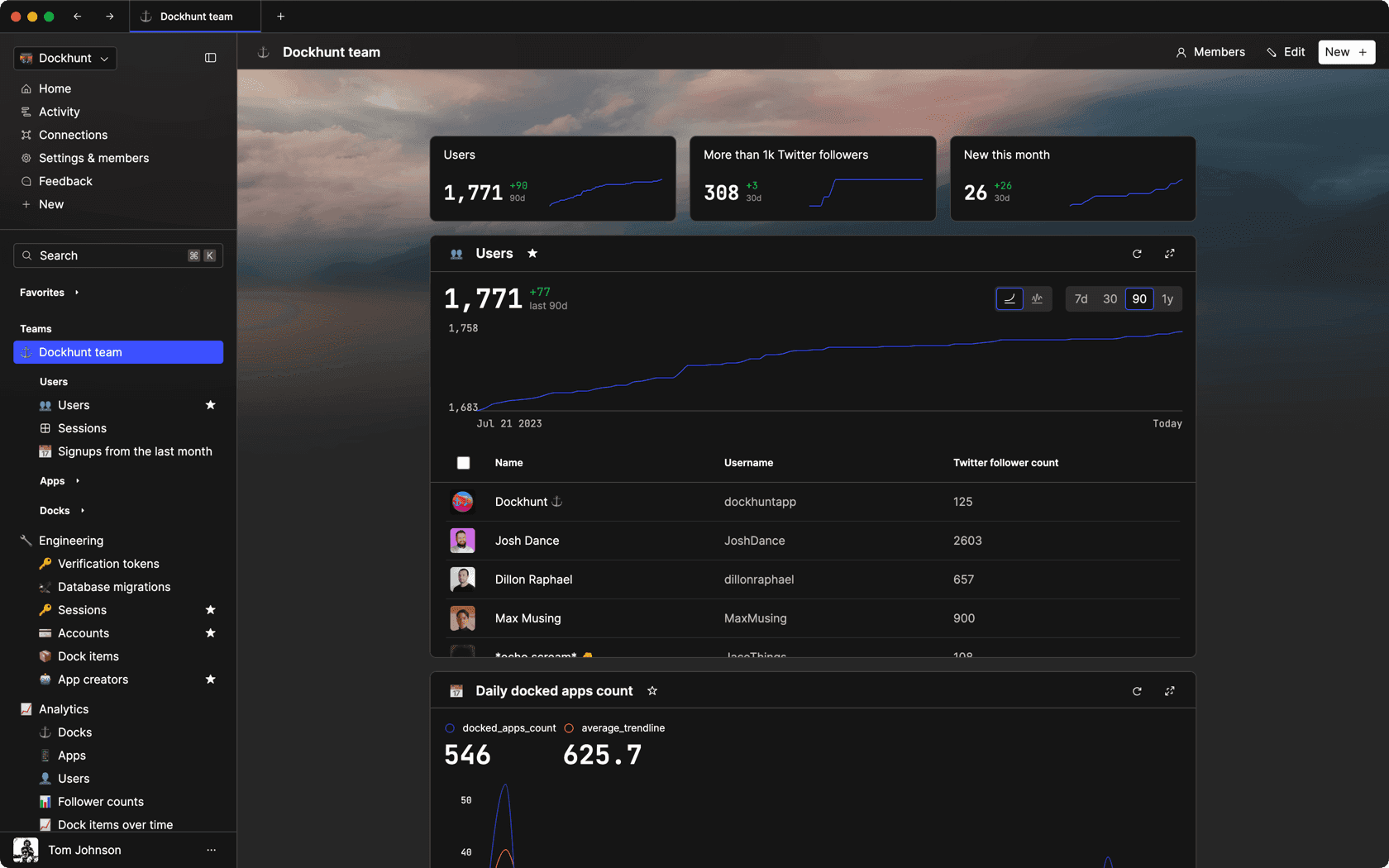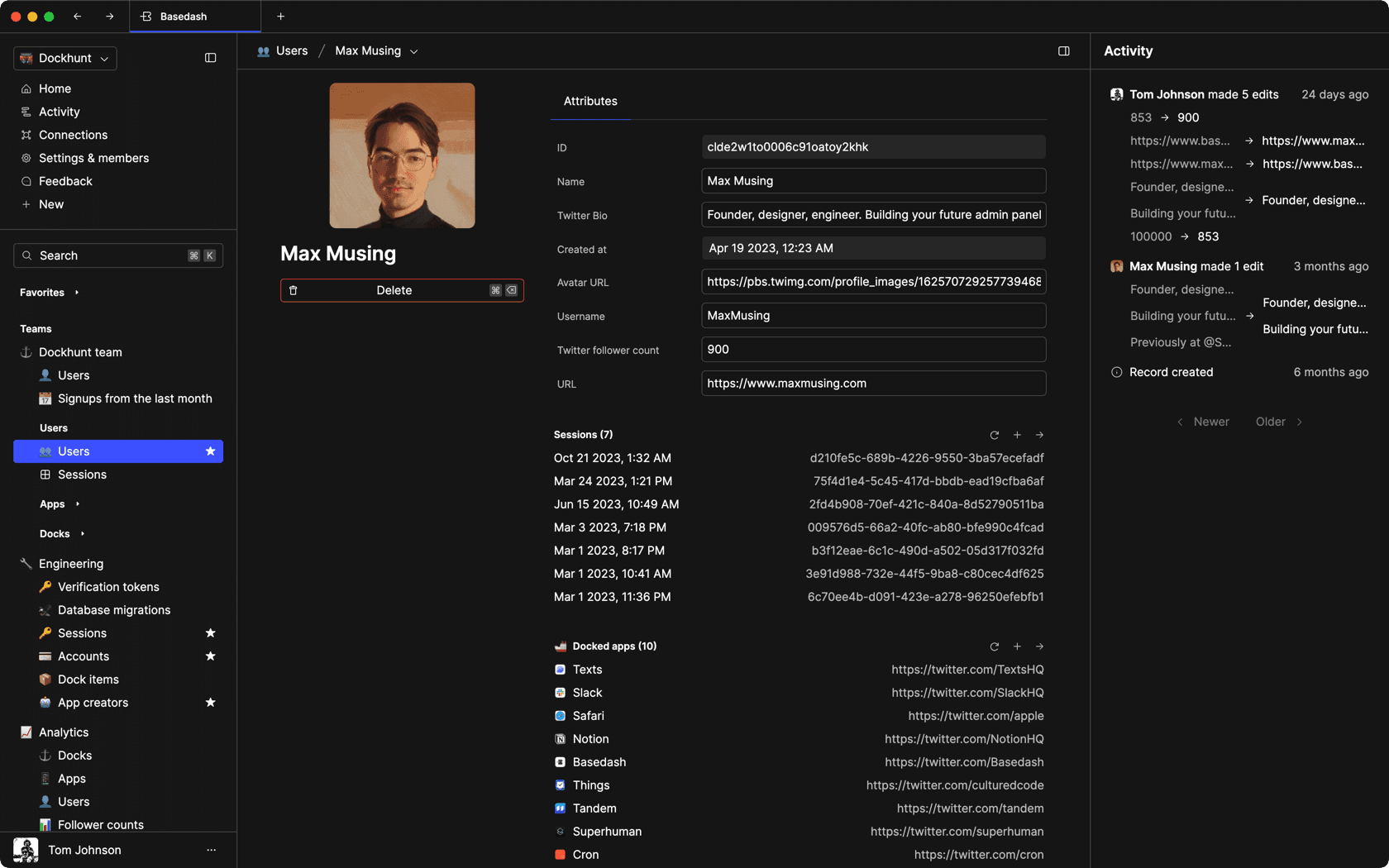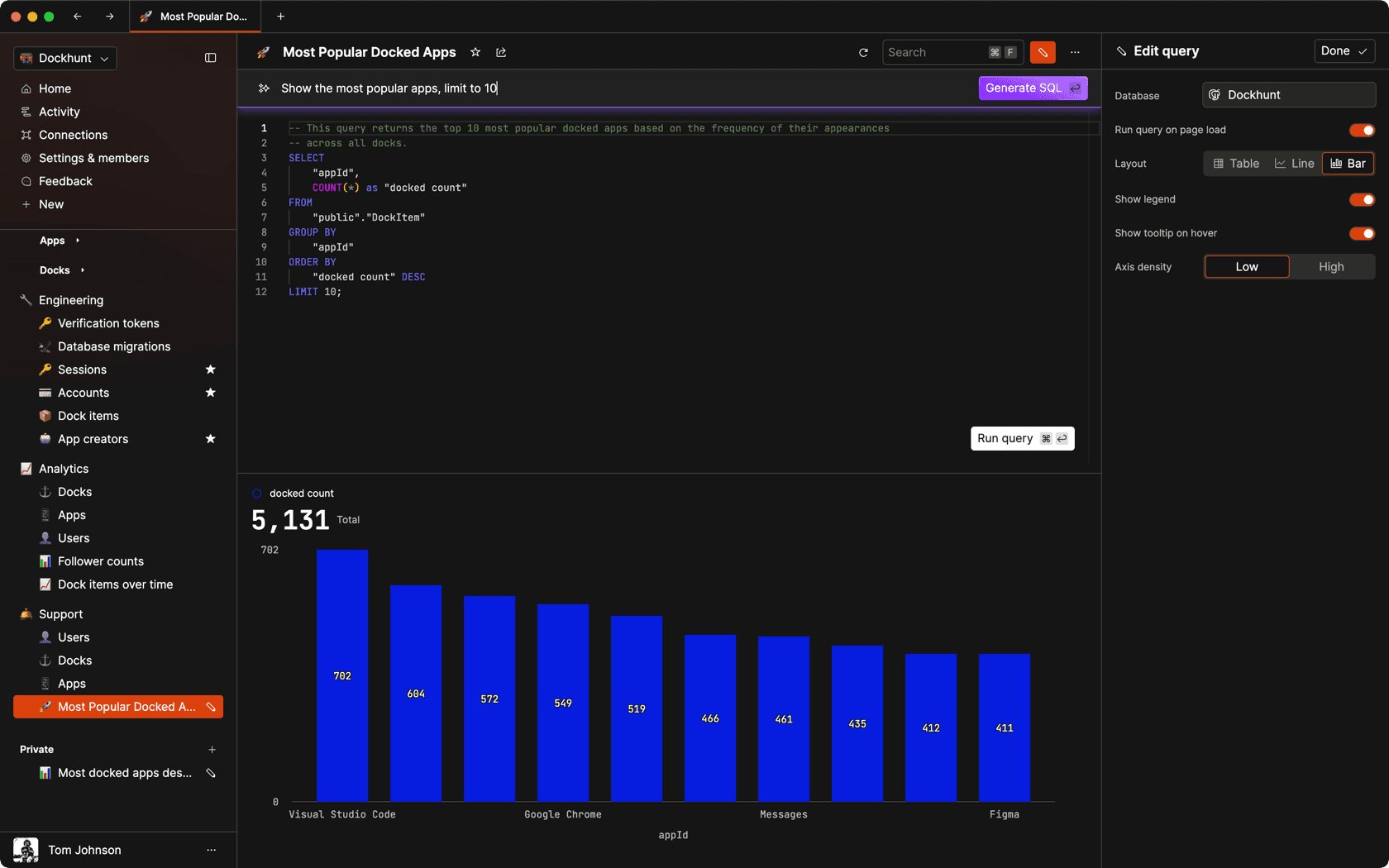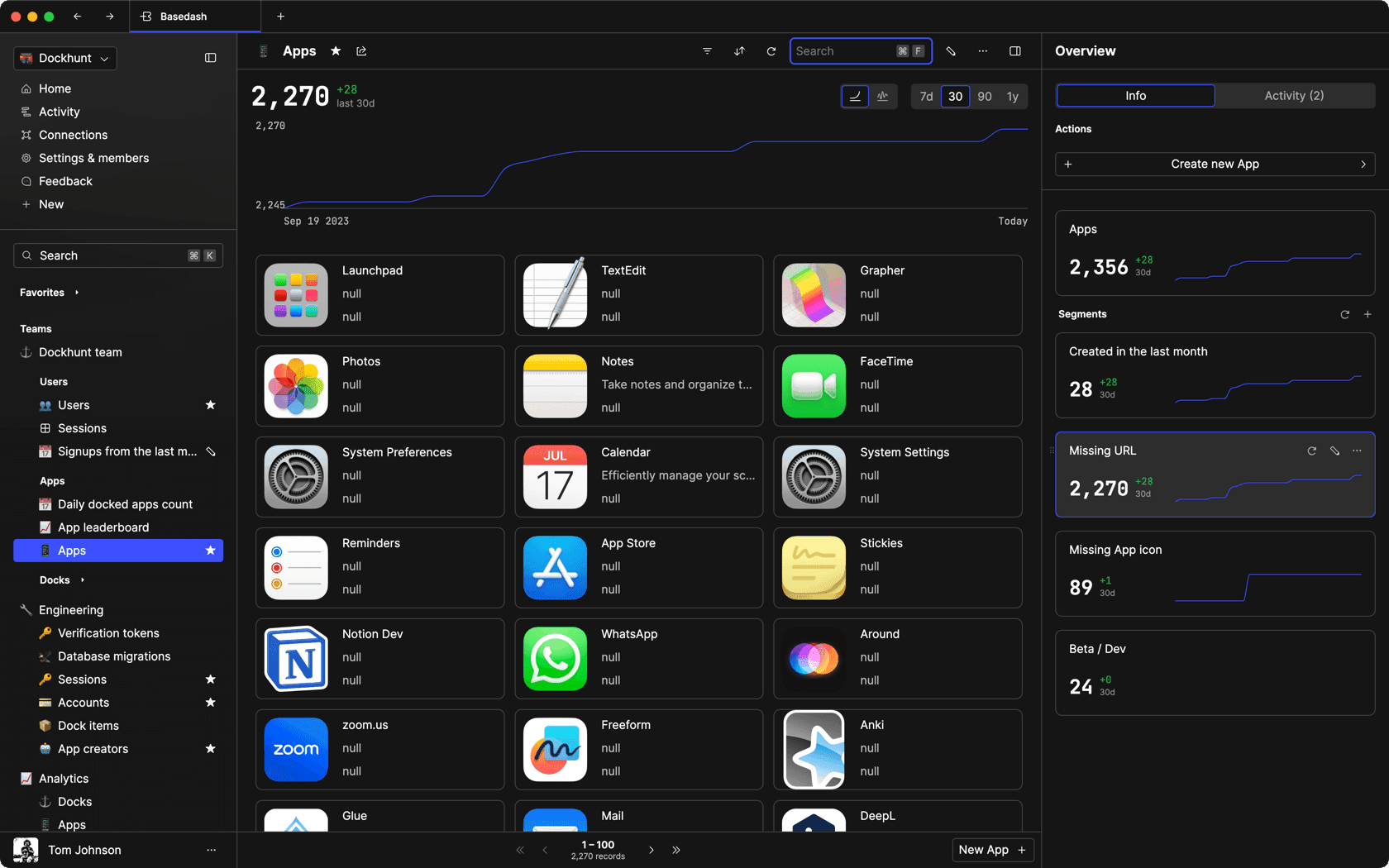### Dashboards and charts

Effortlessly make charts and create a space for your team to work together towards shared goals and metrics.

### SQL composer with AI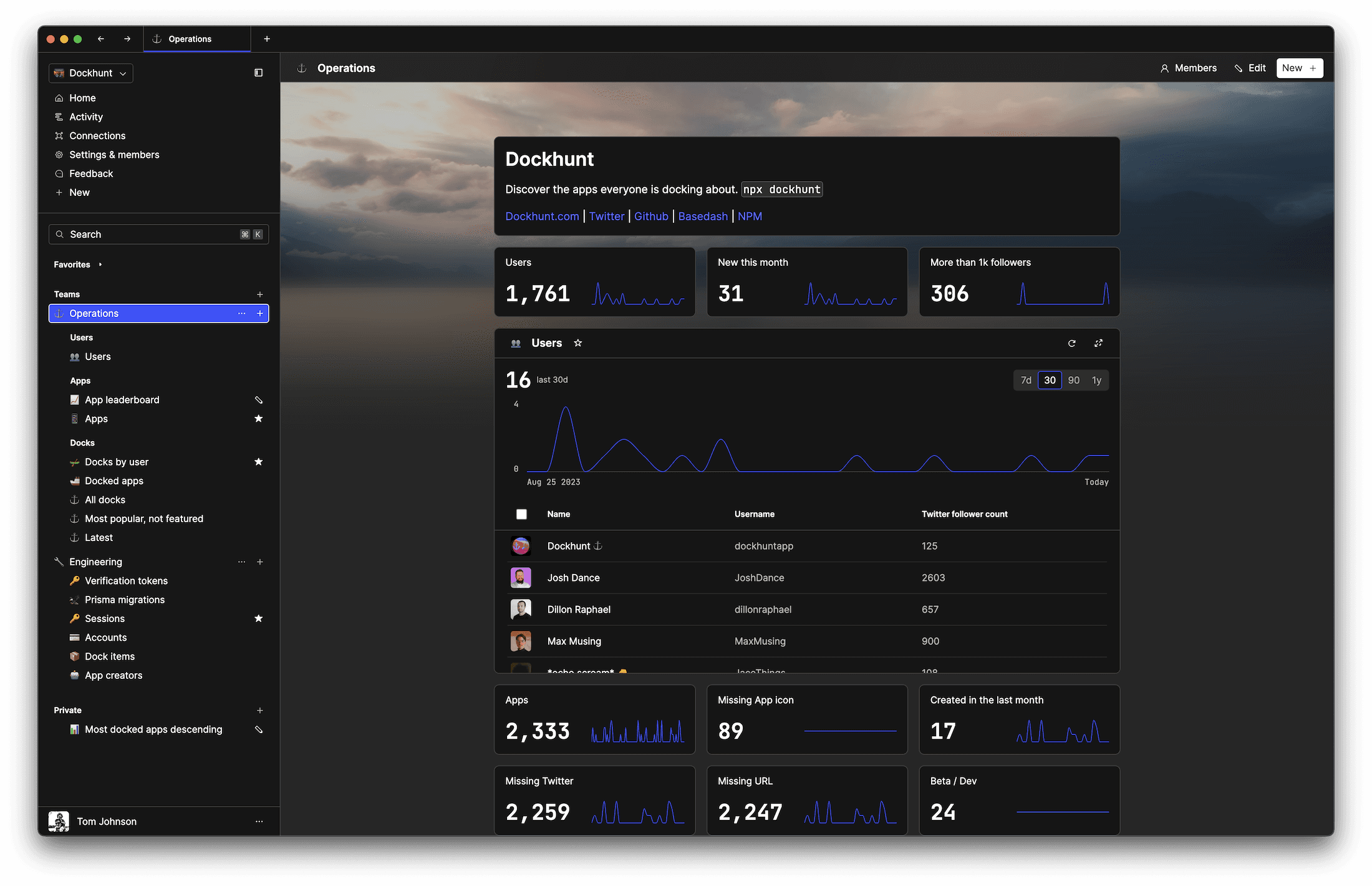SOC II
Type 2 Certified

### Features

Proudly carbon-neutral.SOC II
Type 2 Certified

### Features

Proudly carbon-neutral.SOC II
Type 2 Certified